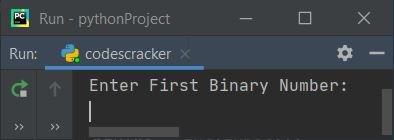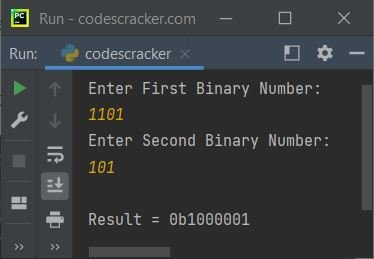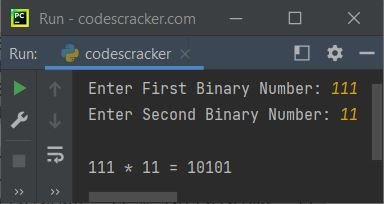# Python Program to Multiply Two Binary Numbers

• Multiply two binary numbers using pre-defined function
• Multiply two binary numbers without using any pre-defined function, rather using user-based or self-used code

## Binary Number Multiplication using Function

The question is, write a Python program that multiplies two binary numbers entered by user at run-time. The upcoming program is answer to this question:

```print("Enter First Binary Number: ")
binOne = int(input())
print("Enter Second Binary Number: ")
binTwo = int(input())

binOne = str(binOne)
binTwo = str(binTwo)

decMul = int(binOne, 2) * int(binTwo, 2)
binMul = bin(decMul)

print("\nResult = " + binMul)```

Here is its sample run:Now supply inputs say 1101 as first and 101 as second binary number, to multiply these two given binary numbers and print the multiplication result on output as shown in the snapshot given below:#### Modified Version of Previous Program

This program is the modified version of previous program. The end used in this program, is to skip insertion of an automatic newline using print(). The str() converts from any type to a string type. The int() method converts into decimal number format.

```print(end="Enter First Binary Number: ")
binOne = int(input())
print(end="Enter Second Binary Number: ")
binTwo = int(input())

binOne = str(binOne)
binTwo = str(binTwo)
decMul = int(binOne, 2) * int(binTwo, 2)
binMul = bin(decMul)

print("\n" + binOne + " * " + binTwo + " = " + binMul[2:])```

Here is its sample run with user input, 111 as first and 11 as second binary number:## Binary Number Multiplication without using Function

This is the main program of this article. Proceeding anything in a programming world using self-created or user-based code is better way to learn the things. That is using any pre-defined function to do the task will not allow you to learn more. Rather it is like remembering the things.

But using or understanding user-based code to do the same task gives you more and deep understanding about the logic, processes, loops, and all that in a better way. So always try to create user-based code for each and every task. Like this program multiplies two binary numbers entered by user, using self-created code by one of the codescracker programmer. Let's have a look at the program and its sample run:

```def binProd(binOne, binTwo):
i = 0
rem = 0
sum = []
bProd = 0
while binOne != 0 or binTwo != 0:
sum.insert(i, (((binOne % 10) + (binTwo % 10) + rem) % 2))
rem = int(((binOne % 10) + (binTwo % 10) + rem) / 2)
binOne = int(binOne/10)
binTwo = int(binTwo/10)
i = i+1
if rem != 0:
sum.insert(i, rem)
i = i+1
i = i-1
while i >= 0:
bProd = (bProd * 10) + sum[i]
i = i-1
return bProd

binMul = 0
factr = 1
print(end="Enter First Binary Number: ")
binOne = int(input())
print(end="Enter Second Binary Number: ")
binTwo = int(input())
while binTwo != 0:
digit =  binTwo % 10
if digit == 1:
binOne = binOne * factr
binMul = binProd(binOne, binMul)
else:
binOne = binOne * factr
binTwo = int(binTwo/10)
factr = 10
print("\nMultiplication Result = " +str(binMul))```

Here is its sample run with user input, 110 as first and 10 as second binary number:Python Online Test

« Previous Program Next Program »